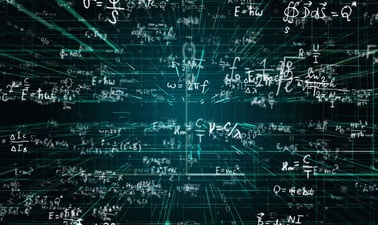# The London School of Economics and Political Science: Mathematics 1 Part 1: Differential Calculus

4.6 stars
7 ratings

The first in a series of two courses from LSE which help you to master mathematics fundamentals and build your quantitative skillset for progression in high-growth careers, or to use as step towards further study at undergraduate level.5 weeks
12–15 hours per week
Self-paced
Free

Mathematics 1 Part 1 is the first of two self-paced courses from LSE which aim to introduce and develop your understanding of essential mathematical concepts, methods and techniques. This course can be taken alone or as part of the LSE MicroBachelors program in Mathematics and Statistics Fundamentals.

Part 1 covers the following topics:

• Functions and graphs

• The derivative

• Curve sketching and optimisation

• Functions of two variables and partial derivatives

• Critical points of two-variable functions

This course will equip you with the skills to solve unseen mathematical problems in these areas and will emphasise their applications to problems in economics, management, and related areas.

### At a glance

• Institution: LSE
• Subject: Math
• Level: Intermediate
• Prerequisites:

Secondary school (high school) algebra; basic knowledge of mathematical concepts. It is strongly reccommeded to study the LSE mathematics courses in order (progressing to Mathematics 1, Part 2) given the cumulative nature of the subject matter.

• Language: English
• Video Transcript: English
• Associated programs:
• Associated skills: Basic Math, Management, Sketching, Differential Calculus, Statistics, Economics

# What you'll learn

Skip What you'll learn

By the end of this course you will have:

• used the concepts, terminology, methods and conventions covered in the course to solve mathematical problems
• the ability to solve unseen mathematical problems involving understanding of these concepts and application of these methods
• seen how mathematical techniques can be used to solve problems in economics and related subjects
• acquired skills in the methods of calculus (including multivariate calculus) and linear algebra, as required for their use in economics-based subjects

# Syllabus

Skip Syllabus
• Functions and graphs
• The derivative
• Curve sketching and optimisation
• Functions of two variables and partial derivatives
• Critical points of two-variable functions
• Final exam